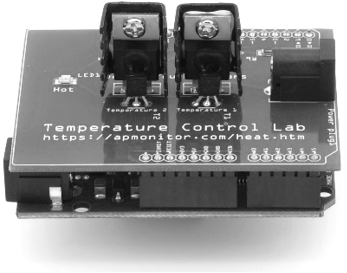## Laplace TransformsLaplace transforms convert a function f(t) in the time domain into function in the Laplace domain F(s).

$$F(s) = \mathcal{L}\left(f(t)\right) = \int_0^\infty f(t)e^{-s\,t}dt$$

As an example of the Laplace transform, consider a constant c. The function f(t) = c and the following expression is integrated.

$$\mathcal{L}(c)=\int_0^\infty c \, e^{-s\,t} dt = -\frac{c}{s}e^{-s\,t} \biggr\rvert_0^\infty = 0 - \left(-\frac{c}{s} \right) = \frac{c}{s}$$

Mathematicians have developed tables of commonly used Laplace transforms. Below is a summary table with a few of the entries that will be most common for analysis of linear differential equations in this course. Notice that the derived value for a constant c is the unit step function with c=1 where a signal output changes from 0 to 1 at time=0.

#### Laplace Transform Table

 f(t) in Time Domain F(s) in Laplace Domain $$\delta(t)\quad \mathrm{unit \; impulse}$$ $$1$$ $$S(t) \quad \mathrm{unit \; step}$$ $$\frac{1}{s}$$ $$t \quad \mathrm{ramp \; with \; slope = 1}$$ $$\frac{1}{s^2}$$ $$t^{n-1}$$ $$\frac{(n-1)!}{s^n}$$ $$e^{-b\,t}$$ $$\frac{1}{s+b}$$ $$1-e^{-t/\tau}$$ $$\frac{1}{s(\tau s + 1)}$$ $$\sin(\omega t)$$ $$\frac{\omega}{s^2+\omega^2}$$ $$\cos(\omega t)$$ $$\frac{s}{s^2+\omega^2}$$ $$\frac{1}{\tau_1-\tau_2}\left(\exp{\left(-t/\tau_1\right)} - \exp{\left(-t/\tau_2 \right)} \right)$$ $$\frac{1}{\left(\tau_1s+1\right)\left(\tau_2s+1\right)}$$ $$\frac{1}{\tau^n \left(n-1\right)!}t^{n-1}\exp{\left(-\frac{t}{\tau}\right)}$$ $$\frac{1}{\left(\tau s+1\right)^n}$$ $$\frac{1}{\tau \sqrt{1-\zeta^2}} \exp{\left(-\frac{\zeta \, t}{\tau}\right)} \sin{ \left( \sqrt{1-\zeta^2} \frac{t}{\tau} \right) }$$ $$\frac{1}{\tau^2 s^2 + 2 \zeta \tau s + 1}$$ See 2nd Order Systems $$\frac{1}{s\left(\tau^2 s^2 + 2 \zeta \tau s + 1\right)}$$ $$\frac{df}{dt}$$ $$sF(s)-f(0)$$ $$\frac{d^nf}{dt^n}$$ $$s^n F(s) - s^{n-1} f(0) - s^{n-2}f^{(1)}(0) - \ldots \\ - sf^{(n-2)}(0) - f^{(n-1)}(0)$$ $$\int f(t)$$ $$\frac{F(s)}{s}$$ $$f\left(t-t_0\right)S\left(t-t_0\right)$$ $$e^{-t_0s}F(s)$$

Note that the functions f(t) and F(s) are defined for time greater than or equal to zero. The next step of transforming a linear differential equation into a transfer function is to reposition the variables to create an input to output representation of a differential equation.

#### Final Value Theorem

The Final Value Theorem (FVT) gives the steady state signal value y_\infty for a stable system. Note that the Laplace variable s is multiplied by the signal Y(s) before the limit is taken.

$$y_\infty = \lim_{s \to 0} s \, Y(s)$$

The FVT may give misleading results if applied to an unstable system. It is only applicable to stable systems or signals. There is more information on how to determine system stability.

#### Initial Value Theorem

The Initial Value Theorem (IVT) gives an initial condition of a signal by taking the limit as s \to \infty. Like the FVT, the Laplace variable s is multiplied by the signal Y(s) before the limit is taken.

$$y_0 = \lim_{s \to \infty} s \, Y(s)$$

#### Laplace Transforms with Python

Python Sympy is a package that has symbolic math functions. A few of the notable ones that are useful for this material are the Laplace transform (laplace_transform), inverse Laplace transform (inverse_laplace_transform), partial fraction expansion (apart), polynomial expansion (expand), and polynomial roots (roots).Sympy for Laplace Transforms (Python Notebook and Google Colab)
import sympy as sym
from sympy.abc import s,t,x,y,z
from sympy.integrals import laplace_transform
from sympy.integrals import inverse_laplace_transform

# Laplace transform (t->s)
U = laplace_transform(5*t, t, s)
print('U')
print(U)
# Result: 5/s**2

# Inverse Laplace transform (s->t)
X = inverse_laplace_transform(U,s,t)
print('X')
print(X)
# Result: 5*t*Heaviside(t)

# Function
F = 5*(s+1)/(s+3)**2
print('F')
print(F)
# Result: (5*s + 5)/(s + 3)**2

# Partial fraction decomposition
G = sym.apart(F)
print('G')
print(G)
# Result: 5/(s + 3) - 10/(s + 3)**2

# denominator of transfer function
d1 = (s+1)*(s+3)*(s**2+3*s+1)

# expand polynomial
d2 = sym.expand(d1)
print('d2')
print(d2)
# Result: s**4 + 7*s**3 + 16*s**2 + 13*s + 3

# find roots
print(sym.roots(d2))
# Result: {-1: 1, -3: 1, -3/2 - sqrt(5)/2: 1, -3/2 + sqrt(5)/2: 1}

See additional Sympy Laplace Transform code in the example with Transfer Functions.